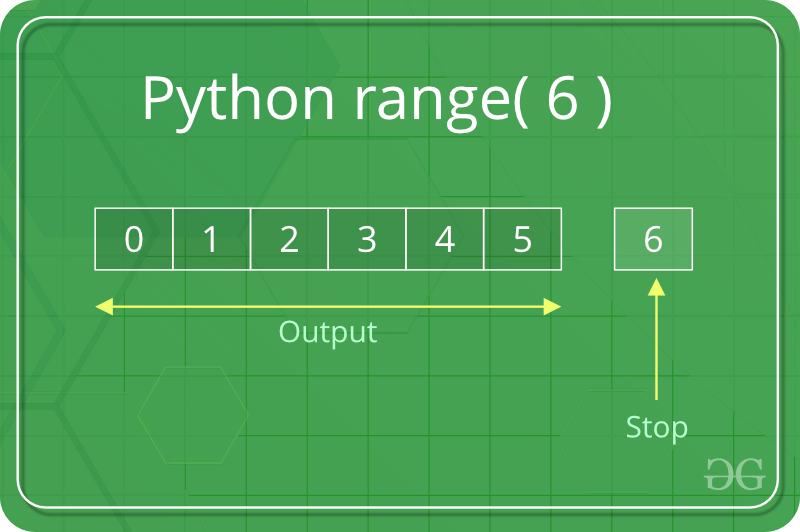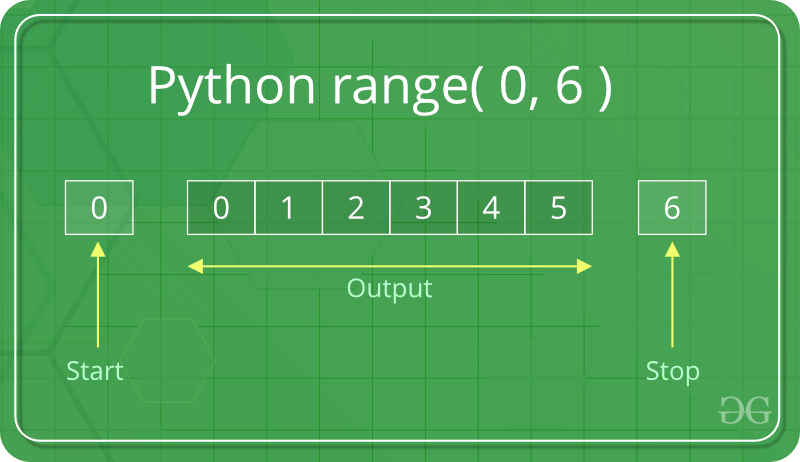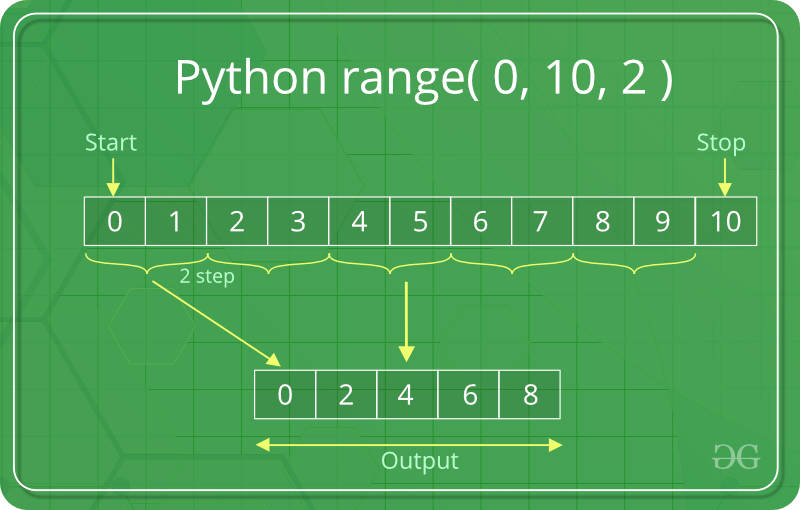# Python range() function

`range()` is a built-in function of Python. It is used when a user needs to perform an action for a specific number of times. `range()` in Python(3.x) is just a renamed version of a function called `xrange `in Python(2.x). The range() function is used to generate a sequence of numbers.

`range()` is commonly used in for looping hence, knowledge of same is key aspect when dealing with any kind of Python code. Most common use of `range()` function in Python is to iterate sequence type (List, string etc.. ) with for and while loop.

Python `range()` Basics :
In simple terms, `range()` allows user to generate a series of numbers within a given range. Depending on how many arguments user is passing to the function, user can decide where that series of numbers will begin and end as well as how big the difference will be between one number and the next.`range()` takes mainly three arguments.

• start: integer starting from which the sequence of integers is to be returned
• stop: integer before which the sequence of integers is to be returned.
The range of integers end at stop – 1.
• step: integer value which determines the increment between each integer in the sequence

 `# Python Program to  ` `# show range() basics ` ` `  `# printing a number ` `for` `i ``in` `range``(``10``): ` `    ``print``(i, end ``=``" "``) ` `print``() ` ` `  `# using range for iteration ` `l ``=` `[``10``, ``20``, ``30``, ``40``] ` `for` `i ``in` `range``(``len``(l)): ` `    ``print``(l[i], end ``=``" "``) ` `print``() ` ` `  `# performing sum of natural ` `# number ` `sum` `=` `0` `for` `i ``in` `range``(``1``, ``11``): ` `    ``sum` `=` `sum` `+` `i ` `print``(``"Sum of first 10 natural number :"``, ``sum``) `

Output :

```0 1 2 3 4 5 6 7 8 9
10 20 30 40
Sum of first 10 natural number : 55
```

There are three ways you can call `range() :`

• `range(stop)` takes one argument.
• `range(start, stop)` takes two arguments.
• `range(start, stop, step)` takes three arguments.

#### range(stop)

When user call `range()` with one argument, user will get a series of numbers that starts at 0 and includes every whole number up to, but not including, the number that user have provided as the stop. For Example –`# Python program to ` `# print whole number ` `# using range() ` ` `  `# printing first 10  ` `# whole number ` `for` `i ``in` `range``(``10``): ` `    ``print``(i, end ``=``" "``) ` `print``() ` ` `  `# printing first 20 ` `# whole number ` `for` `i ``in` `range``(``20``): ` `    ``print``(i, end ``=` `" "``) `

Output:

```0 1 2 3 4 5 6 7 8 9
0 1 2 3 4 5 6 7 8 9 10 11 12 13 14 15 16 17 18 19
```

#### range(start, stop)

When user call range() with two arguments, user get to decide not only where the series of numbers stops but also where it starts, so user don’t have to start at 0 all the time. User can use `range()` to generate a series of numbers from X to Y using a range(X, Y). For Example -arguments`# Python program to  ` `# print natural number ` `# using range ` ` `  `# printing a natural ` `# number upto 20 ` `for` `i ``in` `range``(``1``, ``20``): ` `    ``print``(i, end ``=``" "``) ` `print``() ` ` `  `# printing a natural ` `# number from 5 t0 20 ` `for` `i ``in` `range``(``5``, ``20``): ` `    ``print``(i, end ``=``" "``) `

Output:

```1 2 3 4 5 6 7 8 9 10 11 12 13 14 15 16 17 18 19
5 6 7 8 9 10 11 12 13 14 15 16 17 18 19
```

#### range(start, stop, step)

When user call `range()` with three arguments, user can choose not only where the series of numbers will start and stop but also how big the difference will be between one number and the next. If user don’t provide a step, then range() will automatically behave as if the step is 1.

 `# Python program to  ` `# print all number  ` `# divisible by 3 and 5 ` `  `  `# using range to print number ` `# divisible by 3 ` `for` `i ``in` `range``(``0``, ``30``, ``3``): ` `    ``print``(i, end ``=` `" "``) ` `print``() ` ` `  `# using range to print number ` `# divisible by 5 ` `for`  `i ``in` `range``(``0``, ``50``, ``5``): ` `     ``print``(i, end ``=` `" "``) ` `     `

Output :

```0 3 6 9 12 15 18 21 24 27
0 5 10 15 20 25 30 35 40 45
```In this example, we are printing an even number between 0 to 10 so we choose our starting point from 0(start = 0) and stop the series at 10(stop = 10). For printing even number the difference between one number and the next must be 2 (step = 2) after providing a step we get a following output ( 0, 2, 4, 8).

Incrementing with range using positive step :
If user want to increment, then user need `step` to be a positive number. For example:

 `# Python program to ` `# increment with ` `# range() ` ` `  `# incremented by 2 ` `for` `i ``in` `range``(``2``, ``25``, ``2``): ` `    ``print``(i, end ``=``" "``) ` `print``() ` ` `  `# incremented by 4 ` `for` `i ``in` `range``(``0``, ``30``, ``4``): ` `    ``print``(i, end ``=``" "``) ` `print``() ` ` `  `# incremented by 3 ` `for` `i ``in` `range``(``15``, ``25``, ``3``): ` `    ``print``(i, end ``=``" "``) `

Output :

```2 4 6 8 10 12 14 16 18 20 22 24
0 4 8 12 16 20 24 28
15 18 21 24
```

Decrementing with range using negative step :
If user want to decrement, then user need `step` to be a negative number. For example:

 `# Python program to ` `# decrement with ` `# range() ` ` `  `# incremented by -2 ` `for` `i ``in` `range``(``25``, ``2``, ``-``2``): ` `    ``print``(i, end ``=``" "``) ` `print``() ` ` `  `# incremented by -4 ` `for` `i ``in` `range``(``30``, ``1``, ``-``4``): ` `    ``print``(i, end ``=``" "``) ` `print``() ` ` `  `# incremented by -3 ` `for` `i ``in` `range``(``25``, ``-``6``, ``-``3``): ` `    ``print``(i, end ``=``" "``) `

Output :

```25 23 21 19 17 15 13 11 9 7 5 3
30 26 22 18 14 10 6 2
25 22 19 16 13 10 7 4 1 -2 -5
```

Using float Numbers in Python range() :
Python `range()` function doesn’t support the float numbers. i.e. user cannot use floating-point or non-integer number in any of its argument. User can use only integer numbers.For example

 `# Python program to ` `# show using float ` `# number in range() ` ` `  `# using a float number ` `for` `i ``in` `range``(``3.3``): ` `    ``print``(i) ` ` `  `# using a float number ` `for` `i ``in` `range``(``5.5``): ` `    ``print``(i) `

Output :

```for i in range(3.3):
TypeError: 'float' object cannot be interpreted as an integer
```

#### Concatenation of two range() functions

The result from two `range()` functions can be concatenated by using the `chain()` method of `itertools` module. The `chain()` method is used to print all the values in iterable targets one after another mentioned in its arguments.

 `# Python program to concatenate ` `# the result of two range functions ` ` `  ` `  `from` `itertools ``import` `chain ` ` `  ` `  `# Using chain method ` `print``(``"Concatenating the result"``) ` `res ``=` `chain(``range``(``5``), ``range``(``10``, ``20``, ``2``)) ` ` `  `for` `i ``in` `res: ` `    ``print``(i, end``=``" "``) `

Output:

```Concatenating the result
0 1 2 3 4 10 12 14 16 18
```

#### Accessing range() with index value

A sequence of numbers is returned by the `range()` function as it’s object that can be accessed by its index value. Both positive and negative indexing is supported by its object.

 `# Python program to demonstrate ` `# range function ` ` `  ` `  `ele ``=` `range``(``10``)[``0``] ` `print``(``"First element:"``, ele) ` ` `  `ele ``=` `range``(``10``)[``-``1``] ` `print``(``"\nLast element:"``, ele) ` ` `  `ele ``=` `range``(``10``)[``4``] ` `print``(``"\nFifth element:"``, ele) `

Output:

```First element: 0

Last element: 9

Fifth element: 4
```

Points to remember about Python `range()` function :

• `range()` function only works with the integers i.e. whole numbers.
• All argument must be integers. User can not pass a string or float number or any other type in a start, stop and step argument of a range().
• All three arguments can be positive or negative.
• The step value must not be zero. If a step is zero python raises a ValueError exception.
• `range()` is a type in Python
•  `# Python program to ` `# show range() type ` ` `  `# checking a type of  ` `# range ` `type``(``range``(``3``)) `

• User can access items in a `range()` by index, just as user do with a list:
•  `# Python program to ` `# access items in a range ` ` `  `# accessing a items ` `range``(``3``)[``1``] ` ` `  `# accessing a items ` `range``(``3``)[``2``] `

My Personal Notes arrow_drop_upIf you like GeeksforGeeks and would like to contribute, you can also write an article using contribute.geeksforgeeks.org or mail your article to contribute@geeksforgeeks.org. See your article appearing on the GeeksforGeeks main page and help other Geeks.

Please Improve this article if you find anything incorrect by clicking on the "Improve Article" button below.

Article Tags :

5

Please write to us at contribute@geeksforgeeks.org to report any issue with the above content.